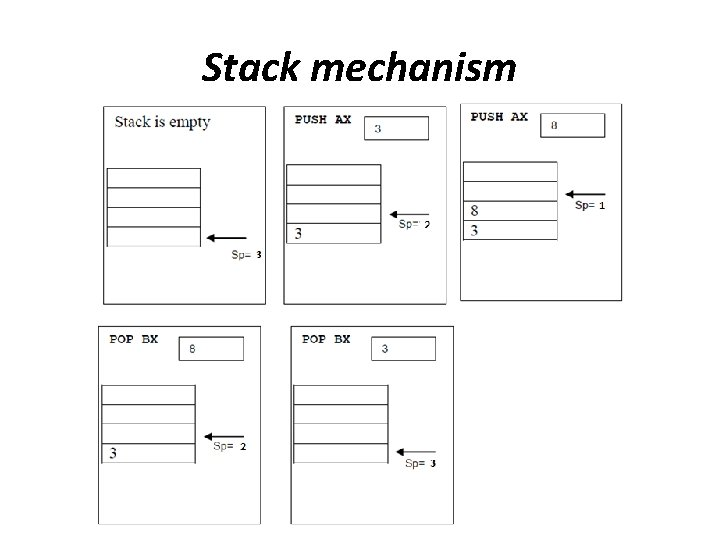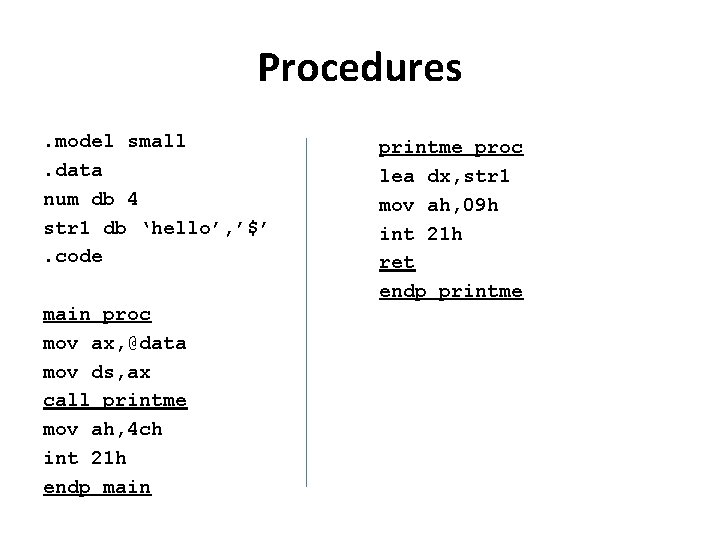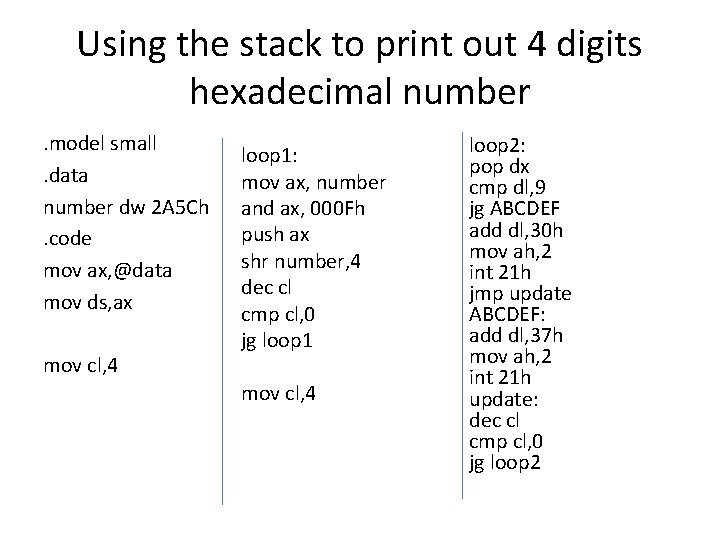# Working with the Stack Stack concept Stack is

• Slides: 9Working with the StackStack concept • Stack is a special memory area used to store data temporarily when calling a subprogram and nested loops. Stack mechanism works in style “Last In First Out” LIFO. The following figure shows the stack mechanism.Stack mechanismPUSH & POP instructions • push REG/MEM 16 -bits only • push ax Store AX in the top of stack memory area then decrement SP register. • pop REG/MEM 16 -bits only • pop bx Increment SP register then copy the value in the top of stack to BX.e. g. using the stack to print an array in reverse way. model small. data x db 0, 1, 2, 3, 4, 5, 6, 7, 8, 9. code mov ax, @data mov ds, ax lea si, x mov cx, 10 lop 1: mov ah, 0 mov al, [si] push ax inc si loop lop 1 mov lop 2: mov pop add int cx, 10 ah, 02 h dx dl, '0' 21 h loop lop 2Procedures. model small. data num db 4 str 1 db ‘hello’, ’\$’. code main proc mov ax, @data mov ds, ax call printme mov ah, 4 ch int 21 h endp main printme proc lea dx, str 1 mov ah, 09 h int 21 h ret endp printmeUsing the stack to print out 4 digits decimal number. model small. data number dw 2153. code mov ax, @data mov ds, ax mov cl, 4 loop 1: mov ax, number mov bl, 10 div bl mov dl, ah mov dh, 0 push dx mov ah, 0 mov number, ax dec cl cmp cl, 0 jg loop 1 mov cl, 4 loop 2: pop dx add dl, 30 h mov ah, 2 int 21 h dec cl cmp cl, 0 jg loop 2Using the stack to print out 4 digits hexadecimal number. model small. data number dw 2 A 5 Ch. code mov ax, @data mov ds, ax mov cl, 4 loop 1: mov ax, number and ax, 000 Fh push ax shr number, 4 dec cl cmp cl, 0 jg loop 1 mov cl, 4 loop 2: pop dx cmp dl, 9 jg ABCDEF add dl, 30 h mov ah, 2 int 21 h jmp update ABCDEF: add dl, 37 h mov ah, 2 int 21 h update: dec cl cmp cl, 0 jg loop 2Question For the following assembly language program, find the content of the register AL after the execution of each underlined instruction: . MODEL SMALL. DATA M DB 8, 7, 8, 3, 2, 9. CODE MOV AX, @data MOV DS, AX LEA BX, M MOV AL, [BX] SHR AL, 2 SHL AL, 1 OR AL, 0 INC BX ADD AL, [BX] ADD BX, 3 SUB AL, [BX] MOV DX, 12 PUSH DX MOV DL, 3 MOV AH, 0 DIV DL MOV DL, 2 MUL DL POP AX MOV AL, '0' MOV AH, 4 ch INT 21 h AL = 8 2 4 4 AL = 11 AL = 9 AL = 4 AL = 8 AL = 12 AL = 30 h